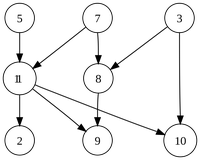# Glossary

## Directed Edge

A directed edge is an edge contained in a directed graph, which means that the edge must also have an orientation, which is represented by using an arrow for the directed edge: the tail and head of this arrow are the nodes representing the beginning and ending points of the edge. We may think of the directed edges as "one-way streets"; when traversing directed edges from node to node, we typically demand that we always travel down an edge in the legal direction (i.e., from its tail to its head).

In undirected graphs, an edge can be represented as $\{v, w\}$ or $\{w, v\}$, as the order of the nodes in the edge is unimportant. However, the directed edge $(v, w)$ implies that $v$ is the edge's tail and $w$ is the edge's head; as such, $(v, w)$ differs from the edge $(w, v)$ having opposite orientation. For example, in the sample directed graph shown below, $(3, 8)$ and $(11, 2)$ are directed edges.We also may consider directed loops, which are edges $(v, v)$ whose head and tail are equal.